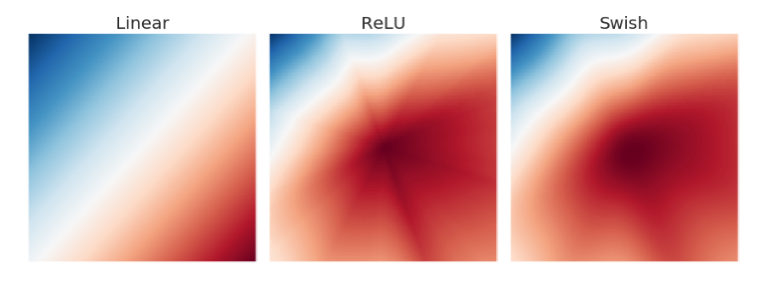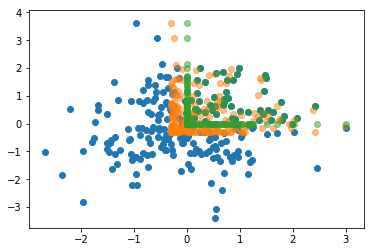The Swish function in PyTorch would be:

def swish(x):
return x * F.sigmoid(x)


The Mish function in PyTorch would be:

def mish(x):
return x * torch.tanh(F.softplus(x))


For both of these functions smoothness plays a beneficial role in optimization and generalization and they achieve a bit better results than ReLU in general.The figure is from the Swish paper, generated by passing two scalars, the x and y into a randomly initialized neural network that outputs a single scalar (the color value).

The ReLU network output landscape has distinctive sharp regions of similar magnitudes whereas the the Swish network output landscape is more smooth.

Interesting may be to compare the regions of data distribution after passing randomly generated normal distributed input to ReLU and Mish in this case.

t = torch.randn(222,222)
plt.scatter(t, t) #blue

m = mish(t)
plt.scatter(m, m, alpha=0.5) #orange

r = F.relu(t)
plt.scatter(r, r, alpha=0.5) #green